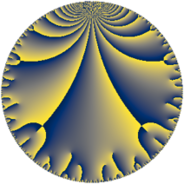# Properties

 Label 91.8.uLevel $91$ Weight $8$ Character orbit 91.u Rep. character $\chi_{91}(30,\cdot)$ Character field $\Q(\zeta_{6})$ Dimension $126$ Newform subspaces $1$ Sturm bound $74$ Trace bound $0$

# Related objects

## Defining parameters

 Level: $$N$$ $$=$$ $$91 = 7 \cdot 13$$ Weight: $$k$$ $$=$$ $$8$$ Character orbit: $$[\chi]$$ $$=$$ 91.u (of order $$6$$ and degree $$2$$) Character conductor: $$\operatorname{cond}(\chi)$$ $$=$$ $$91$$ Character field: $$\Q(\zeta_{6})$$ Newform subspaces: $$1$$ Sturm bound: $$74$$ Trace bound: $$0$$

## Dimensions

The following table gives the dimensions of various subspaces of $$M_{8}(91, [\chi])$$.

Total New Old
Modular forms 134 134 0
Cusp forms 126 126 0
Eisenstein series 8 8 0

## Trace form

 $$126 q - 3 q^{2} - 164 q^{3} + 3841 q^{4} - 6 q^{6} + 754 q^{7} + 85710 q^{9} + O(q^{10})$$ $$126 q - 3 q^{2} - 164 q^{3} + 3841 q^{4} - 6 q^{6} + 754 q^{7} + 85710 q^{9} + 7486 q^{10} - 18328 q^{12} + 1816 q^{13} - 6668 q^{14} - 11817 q^{15} - 237219 q^{16} - 28969 q^{17} + 75423 q^{18} + 12342 q^{20} - 54000 q^{21} + 51388 q^{22} + 17067 q^{23} + 810665 q^{25} + 144300 q^{26} - 528170 q^{27} + 18464 q^{28} + 191096 q^{29} - 432160 q^{30} - 259995 q^{31} + 49149 q^{32} + 87303 q^{35} + 1965131 q^{36} - 571857 q^{37} - 1061954 q^{38} - 1352875 q^{39} + 345362 q^{40} - 636567 q^{41} - 3473694 q^{42} - 659976 q^{43} + 2519268 q^{44} + 1980717 q^{45} - 4068600 q^{46} - 294840 q^{47} + 4726652 q^{48} + 541120 q^{49} - 2908992 q^{50} - 502094 q^{51} + 7809224 q^{52} - 434723 q^{53} + 2119563 q^{54} + 2152196 q^{55} - 858015 q^{56} + 1376949 q^{59} - 3768141 q^{60} - 4854916 q^{61} - 1713436 q^{62} + 10091940 q^{63} - 40270472 q^{64} - 4876182 q^{65} + 8270835 q^{66} + 531379 q^{68} - 311689 q^{69} + 5298534 q^{70} + 1505877 q^{71} - 13207461 q^{73} + 3730527 q^{74} + 5624951 q^{75} + 25297146 q^{76} + 11107478 q^{77} - 9362803 q^{78} + 5012226 q^{79} + 45989766 q^{81} - 34046788 q^{82} - 35428032 q^{84} + 19854117 q^{85} + 5226210 q^{86} + 17331733 q^{87} + 24348310 q^{88} - 43738596 q^{89} + 90517324 q^{90} + 9582031 q^{91} - 8502078 q^{92} - 30517344 q^{93} - 7710416 q^{94} + 14810909 q^{95} + 41499936 q^{96} - 60232128 q^{97} - 65830509 q^{98} + O(q^{100})$$

## Decomposition of $$S_{8}^{\mathrm{new}}(91, [\chi])$$ into newform subspaces

Label Dim $A$ Field CM Traces $q$-expansion
$a_{2}$ $a_{3}$ $a_{5}$ $a_{7}$
91.8.u.a $126$ $28.427$ None $$-3$$ $$-164$$ $$0$$ $$754$$